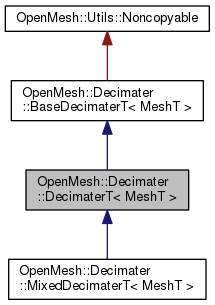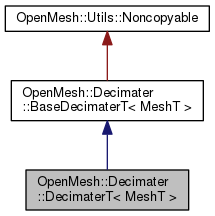OpenMesh
OpenMesh::Decimater::DecimaterT< MeshT > Class Template Reference

Decimater framework. More...

`#include <OpenMesh/Tools/Decimater/DecimaterT.hh>`

Inheritance diagram for OpenMesh::Decimater::DecimaterT< MeshT >:[legend]
Collaboration diagram for OpenMesh::Decimater::DecimaterT< MeshT >:[legend]

## Classes

class  HeapInterface
Heap interface. More...

## Public Types

typedef DecimaterT< MeshT > Self

typedef MeshT Mesh

typedef CollapseInfoT< MeshT > CollapseInfo

typedef ModBaseT< MeshT > Module

typedef std::vector< Module * > ModuleList

typedef ModuleList::iterator ModuleListIterator

typedef Mesh::VertexHandle VertexHandle

typedef Mesh::HalfedgeHandle HalfedgeHandle

typedef Utils::HeapT< VertexHandle, HeapInterfaceDeciHeapPublic Types inherited from OpenMesh::Decimater::BaseDecimaterT< MeshT >
typedef BaseDecimaterT< MeshT > Self

typedef MeshT Mesh

typedef CollapseInfoT< MeshT > CollapseInfo

typedef ModBaseT< MeshT > Module

typedef std::vector< Module * > ModuleList

typedef ModuleList::iterator ModuleListIterator

## Public Member Functions

DecimaterT (Mesh &_mesh)
Constructor.

~DecimaterT ()
Destructor.

size_t decimate (size_t _n_collapses=0)
Perform a number of collapses on the mesh. More...

size_t decimate_to (size_t _n_vertices)
Decimate the mesh to a desired target vertex complexity. More...

size_t decimate_to_faces (size_t _n_vertices=0, size_t _n_faces=0)
Attempts to decimate the mesh until a desired vertex or face complexity is achieved. More...Public Member Functions inherited from OpenMesh::Decimater::BaseDecimaterT< MeshT >
BaseDecimaterT (Mesh &_mesh)

bool initialize ()
Initialize decimater and decimating modules. More...

bool is_initialized () const
Returns whether decimater has been successfully initialized.

void info (std::ostream &_os)
Print information about modules to _os.

void set_observer (Observer *_o)

Observerobserver ()
Get current observer of a decimater.

Mesh & mesh ()
access mesh. used in modules.

template<typename _Module >
bool add (ModHandleT< _Module > &_mh)

template<typename _Module >
bool remove (ModHandleT< _Module > &_mh)
remove module

template<typename Module >
Modulemodule (ModHandleT< Module > &_mh)
get module referenced by handle _mhProtected Member Functions inherited from OpenMesh::Decimater::BaseDecimaterT< MeshT >
bool notify_observer (size_t _n_collapses)
returns false, if abort requested by observer

void set_uninitialized ()
Reset the initialized flag, and clear the bmodules_ and cmodule_.

void update_modules (CollapseInfo &_ci)

bool is_collapse_legal (const CollapseInfo &_ci)
Is an edge collapse legal? Performs topological test only. More...

float collapse_priority (const CollapseInfo &_ci)
Calculate priority of an halfedge collapse (using the modules)

void preprocess_collapse (CollapseInfo &_ci)
Pre-process a collapse.

void postprocess_collapse (CollapseInfo &_ci)
Post-process a collapse.

void set_error_tolerance_factor (double _factor)
This provides a function that allows the setting of a percentage of the original constraint of the modules. More...

void reset ()
Reset the status of this class. More...

## Detailed Description

### template<typename MeshT> class OpenMesh::Decimater::DecimaterT< MeshT >

Decimater framework.

BaseModT, Mesh Decimation Framework

## Member Function Documentation

template<class Mesh >
 size_t OpenMesh::Decimater::DecimaterT< Mesh >::decimate ( size_t _n_collapses = `0` )

Perform a number of collapses on the mesh.

Parameters
 _n_collapses Desired number of collapses. If zero (default), attempt to do as many collapses as possible.
Returns
Number of collapses that were actually performed.
Note
This operation only marks the removed mesh elements for deletion. In order to actually remove the decimated elements from the mesh, a subsequent call to ArrayKernel::garbage_collection() is required.
template<typename MeshT >
 size_t OpenMesh::Decimater::DecimaterT< MeshT >::decimate_to ( size_t _n_vertices )
inline

Decimate the mesh to a desired target vertex complexity.

Parameters
 _n_vertices Target complexity, i.e. desired number of remaining vertices after decimation.
Returns
Number of collapses that were actually performed.
Note
This operation only marks the removed mesh elements for deletion. In order to actually remove the decimated elements from the mesh, a subsequent call to ArrayKernel::garbage_collection() is required.
template<class Mesh >
 size_t OpenMesh::Decimater::DecimaterT< Mesh >::decimate_to_faces ( size_t _n_vertices = `0`, size_t _n_faces = `0` )

Attempts to decimate the mesh until a desired vertex or face complexity is achieved.

Parameters
 _n_vertices Target vertex complexity. _n_faces Target face complexity.
Returns
Number of collapses that were actually performed.
Note
Decimation stops as soon as either one of the two complexity bounds is satisfied.
This operation only marks the removed mesh elements for deletion. In order to actually remove the decimated elements from the mesh, a subsequent call to ArrayKernel::garbage_collection() is required.

The documentation for this class was generated from the following files:

Project OpenMesh, ©  Computer Graphics Group, RWTH Aachen. Documentation generated using doxygen .